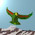## Sunday, September 25, 2011

### Theory Update 114

The other formula given by number 26 uses $\alpha$, the fine structure constant, starting from the observation that $\alpha^{-1}$ is close to $137$. Many have attempted derivations of the modern non integral value for $\alpha$ using the integer $137$. For instance, the Relativist James G. Gilson discusses the beautiful formula

$\alpha = \frac{\cos (\pi /137)}{137}$

noting that other authors have expressed $137$ as $2^7 + 2^3 + 2^0$, as used by number 26. The integer $163 = 137 + 26$ is a favourite Almost Integer known as Ramanujan's constant. It satisfies

$e^{\pi \sqrt{163}} \simeq 640320^3 + 744$

where the decomposition of the large integer is well known in moonshine circles. Alternatively, we can write

$e^{\pi \sqrt{163}} \simeq (C e^{\pi i/24})^{24} - 24$

for $C = \eta (z) / \eta (2z)$, where $z = (1 + \sqrt{163} i)/2$ and $\eta$ is the Dedekind eta function. The $j$-invariant also appears in interesting studies of CFTs, with central charge $48$. Pundits call all this numerology, but mathematicians most certainly do not. Long live the bosonic string.1.So the $3$ comes from the quaternions and the $7$ from the octonions, giving the $10$ dimensions of the superstring. That is, we could also write $163 = 136 + 27$, where $136 = 2^7 + 2^3$ and of course $27 = 3^3$. Qubits and qutrits.

2.Gilson corrects the simple formula to obtain the experimental value for $\alpha$ with the aid of a second integer, which happens to be $26$. This number is supposed to characterise Nature's difficulty in winding fields about a circle. In his paper, he cannot find any reason to consider the number $26$ significant. Maybe he will change his mind.

3.Recall that $7$ qubits show up in Duff and Ferrara's paper on tripartite entanglement and the Fano plane, where the Fano plane is often associated to the $7$ octonions. The $3$ qubits are associated as usual to the $S$,$T$,$U$ dualities. The $3$ qutrits are the tetractys.

4.Don't forget the charged leptons and up-type quarks form a Fano plane when the angle is pi/2 instead of 2/9.

5.Good point, Dave. Actually, M theory has gazillions of these small finite structures floating around in it!

6.The 'near integers' are certainly worth investigating.

Here is a mathematical structure using the 'near integers' which outputs the 'fine structure constant' very well within the newly published Codata 2010 values from NIST.

1500625 e^(4 sqrt(58) pi))/(961 (1+sqrt(2/2396291527))^4)(4/d^4)
((((e^(2pi*sqrt(163)) 70^2)^(1/65536))-1)^-1)^(1/2048) =
808017424794512875886459904961710757005754368000000000

We get for output:

d = 0.007297352568841518513443583197914260254...

Its inverse:

d^-1 = 137.03599909230556307630857983284702577811...

Compare this to Gabrielse's 2008 combined empirical QED
determination or the new Codata 2010: alpha =
0.007297352569(5)

However, there is an arbritrary construct in this and that is 1500625 e^(4 sqrt(58) pi))/(961 (1+sqrt(2/2396291527))^4 This needs to be corrected as a full 'number theoretic' value which will be very close to the Planck mass^2/electron mass^2 squared value if this is correct and solved.

Also, there is a direct physics form for this:

(4/d^4)(a^2/c^2)((((2a^2/b^2)^1/65536)-1)^-1)^1/2048 = very close to
the Monster group integer

Where a = M_pl (Planck mass), b = m_n (neutron mass), c = m_e
(electron mass), d = alpha (fine structure constant) (all values
Codata)

This is an approximation with empirical data but is probably exactly
equivalent to the 'number theoretic form'

The new 2010 Codata vs. the old 2006 Codata is a even a better fit for all of this.

7.Mark, please use dollar signs for inline latex. Nobody can actually read these text expressions. Let me try the first line:

$1500625 \exp(4 \sqrt{58} \pi)/(961 (1+ \sqrt{2/2396291527})^4)(4 /d^4)$
$\times ((((\exp(2 \pi \sqrt{163}) 70^2)^{1/65536} -1)^{-1})^{1/2048}$
$= 808017424794512875886459904961710757005754368000000000$

8.Ugh, I give up.

9.Aside from what is above, here is something very interesting about $e^{\pi \sqrt{163}}$.
If you divide this by 24 you obtain a 'near integer' that is very close to a prime and it has thirteen nines in the tail which makes it a better 'near integer'. There are more examples of these and they involve dividing by 8 or 24.

$\frac{e^{\pi \sqrt{163}}}{24}= = 10939058860032030.999999999999968...$ This is almost the prime 10939058860032031

Some other examples are:
$\frac{e^{\pi \sqrt{43}}}{24}= 36864030.9999907...$ This is almost the prime 36864031
$\frac{e^{\pi \sqrt{67}}}{24}= 6133248030.999999944...$ This is almost the prime 6133248031
$\frac{e^{\pi \sqrt{58}}}{8}= 3073907218.999999977...$ this is almost the prime 3073907219
$\frac{e^{\pi \sqrt{5}}}{8}= 829452.999917...$ This is almost the prime 829453
$\frac{e^{\pi \sqrt{22}}}{8}= 313618.99978...$ This is almost the prime 313619

Next time Kea I will pay more attention to your rules.

10.So Heegner numbers, I see. Yes, interesting, Mark.

Note: Only a member of this blog may post a comment.